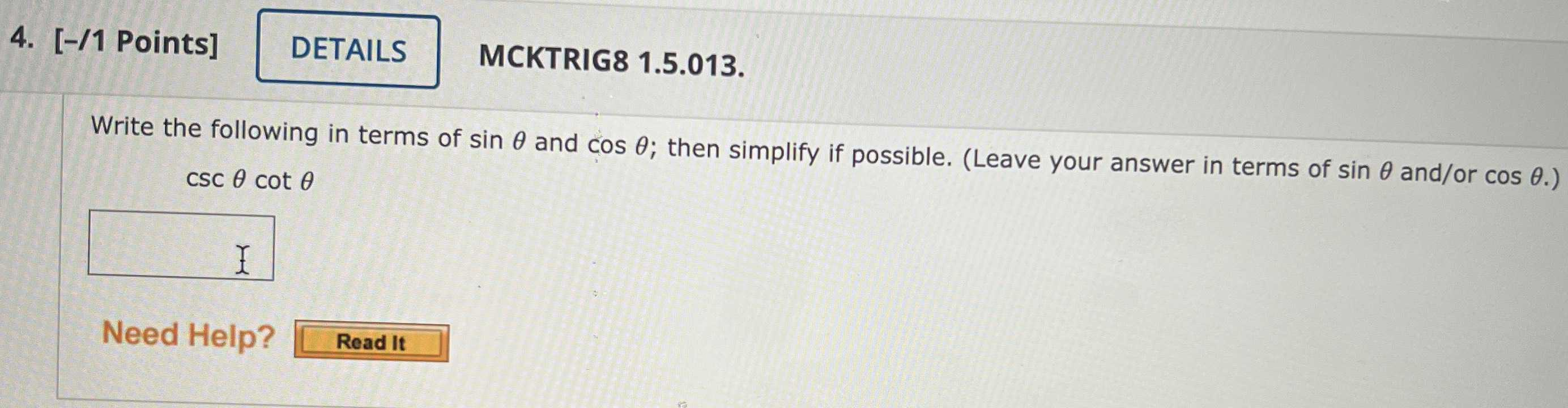### Still have math questions?

Trigonometry
QuestionWrite the following in terms of $$\sin \theta$$ and $$\cos \theta$$ ; then simplify if possible. (Leave your answer in terms of $$\sin \theta$$ and/or $$\cos \theta$$ .)

$$\csc \theta \cot \theta$$

csc$$\theta$$ = 1 / sin$$\theta$$
cot$$\theta$$ = cos$$\theta$$ / sin$$\theta$$
csc$$\theta$$ cot$$\theta$$  = ( 1 / sin$$\theta$$) (cos$$\theta$$ / sin$$\theta$$  )
= (cos$$\theta$$ / sin$$^ { 2}$$ $$\theta$$)